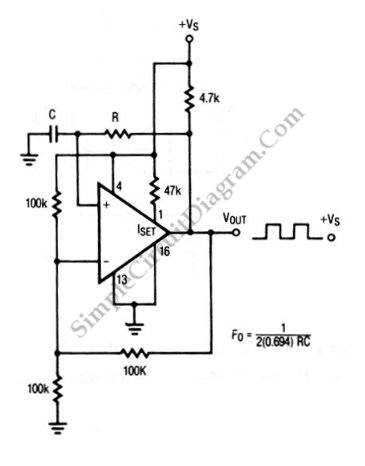# Square Wave Oscillator using Comparator/Op-Amp

The circuit shown in this schematic diagram is a square-wave oscillator circuit.  The main component of this oscillator circuit is LP165/365 comparator. As timing component, it uses capacitor and resistor to determine the frequency operation, generating square waves signal. Here is the schematic diagram of the circuit:We can change the values of R and C to produce different frequencies. The output frequency fo is determined by following equation:
fo=1/(2*(0.649)*R*C